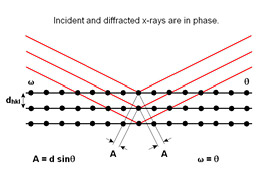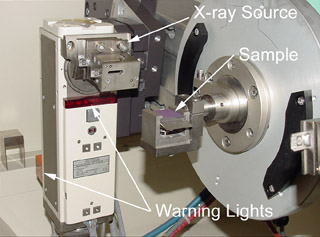# SAMS - X-ray DiffractionThe reflection analogy helps illustrate the geometric requirements of x-ray diffraction

Abstract: This exercise gives the student the chance to plot and analyze x-ray powder diffraction data. The students import the data and plot it, then they determine the structure by matching the diffraction pattern with the reflection rules and the lattice parameters with atomic radii.

This exercise begins with a review of Bragg's law as an equation that is used to predict the positions of diffraction peaks and the reflection rules as a tool for predicting which peaks will actually be present.  It then asks the student to import the data, convert measured intensities to relative intensities, and then plot the data.  Once the data are plotted, peak positions are identified, tabulated, and then analyzed to see which reflection rules apply and what the lattice parameter is.  With this information, the student should be able to identify the substance.

There is an advanced version of this exercise that goes into more depth on this subject. In addition to Bragg's Law and the reflection rules, it also covers a number of the factors that determine the diffraction intensity (multiplicity factors, Lorentz-polarization factor, adsorption, and others). It also includes exercises where the students calculate the diffraction angles and any inter-planar spacing for any crystal system, the structure factor for any peak in any structure, and it all ends by calculating a diffraction pattern.

Prerequisites: familiarity with the nature of x-rays, crystal structures, fundamentals of x-ray diffraction.

Spreadsheet Skills: importing data, separating the imported data into columns, basic formulas, creating charts. This exercise require more in term of planning and organization than they do any advanced spreadsheet techniques.

Download: Click the links below to open the module and the data, or right-click and "Save As..." to copy them to your computer.A view of the x-ray source and sample holder in a powder diffractometer.